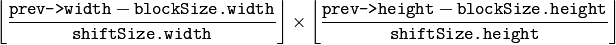# Motion Analysis¶

## CalcOpticalFlowBM¶

Calculates the optical flow for two images by using the block matching method.

C: void cvCalcOpticalFlowBM(const CvArr* prev, const CvArr* curr, CvSize blockSize, CvSize shiftSize, CvSize maxRange, int usePrevious, CvArr* velx, CvArr* vely)
Python: cv.CalcOpticalFlowBM(prev, curr, blockSize, shiftSize, maxRange, usePrevious, velx, vely) → None
Parameters: prev – First image, 8-bit, single-channel curr – Second image, 8-bit, single-channel blockSize – Size of basic blocks that are compared shiftSize – Block coordinate increments maxRange – Size of the scanned neighborhood in pixels around the block usePrevious – Flag that specifies whether to use the input velocity as initial approximations or not. velx – Horizontal component of the optical flow ofsize, 32-bit floating-point, single-channel vely – Vertical component of the optical flow of the same size velx , 32-bit floating-point, single-channel

The function calculates the optical flow for overlapped blocks blockSize.width x blockSize.height pixels each, thus the velocity fields are smaller than the original images. For every block in prev the functions tries to find a similar block in curr in some neighborhood of the original block or shifted by (velx(x0,y0), vely(x0,y0)) block as has been calculated by previous function call (if usePrevious=1)

## CalcOpticalFlowHS¶

Calculates the optical flow for two images using Horn-Schunck algorithm.

C: void cvCalcOpticalFlowHS(const CvArr* prev, const CvArr* curr, int usePrevious, CvArr* velx, CvArr* vely, double lambda, CvTermCriteria criteria)
Python: cv.CalcOpticalFlowHS(prev, curr, usePrevious, velx, vely, lambda, criteria) → None
Parameters: prev – First image, 8-bit, single-channel curr – Second image, 8-bit, single-channel usePrevious – Flag that specifies whether to use the input velocity as initial approximations or not. velx – Horizontal component of the optical flow of the same size as input images, 32-bit floating-point, single-channel vely – Vertical component of the optical flow of the same size as input images, 32-bit floating-point, single-channel lambda – Smoothness weight. The larger it is, the smoother optical flow map you get. criteria – Criteria of termination of velocity computing

The function computes the flow for every pixel of the first input image using the Horn and Schunck algorithm [Horn81]. The function is obsolete. To track sparse features, use calcOpticalFlowPyrLK(). To track all the pixels, use calcOpticalFlowFarneback().

## CalcOpticalFlowLK¶

Calculates the optical flow for two images using Lucas-Kanade algorithm.

C: void cvCalcOpticalFlowLK(const CvArr* prev, const CvArr* curr, CvSize winSize, CvArr* velx, CvArr* vely)
Python: cv.CalcOpticalFlowLK(prev, curr, winSize, velx, vely) → None
Parameters: prev – First image, 8-bit, single-channel curr – Second image, 8-bit, single-channel winSize – Size of the averaging window used for grouping pixels velx – Horizontal component of the optical flow of the same size as input images, 32-bit floating-point, single-channel vely – Vertical component of the optical flow of the same size as input images, 32-bit floating-point, single-channel

The function computes the flow for every pixel of the first input image using the Lucas and Kanade algorithm [Lucas81]. The function is obsolete. To track sparse features, use calcOpticalFlowPyrLK(). To track all the pixels, use calcOpticalFlowFarneback().